# Broad Data Analysis Child Outcome Data Broad Analysis

• Slides: 37
Download presentation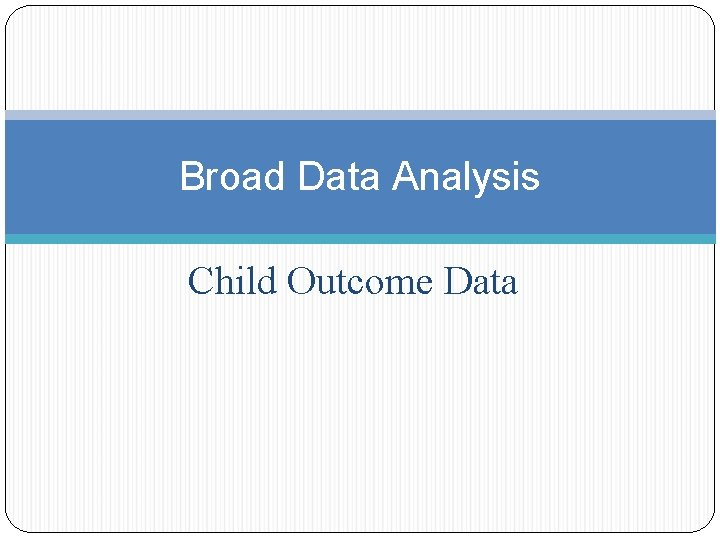Broad Data Analysis Child Outcome DataBroad Analysis: Child Outcomes Does our state’s data look different than the national data? Are our state child outcomes trends stable over time? Is the data trending upwards? Is the data trending downwards? Is our state performing more poorly in some outcomes than others? Are the child outcomes similar across programs? What about data quality? Can we be confident in our data?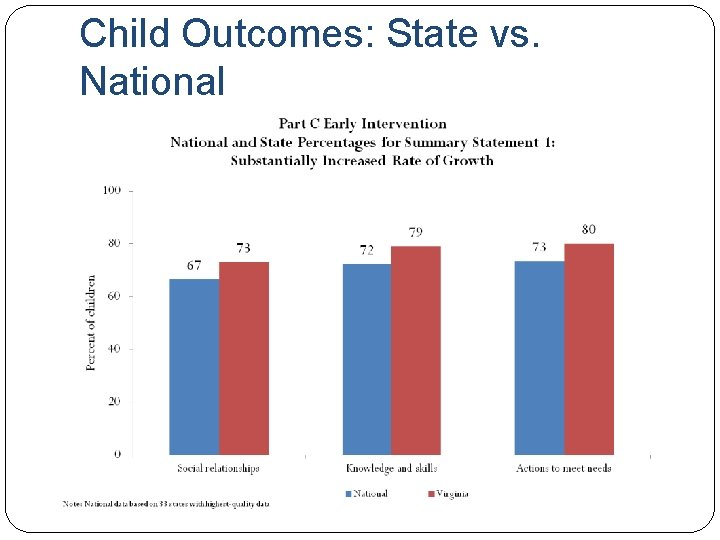Child Outcomes: State vs. NationalChild Outcomes: State vs. NationalNational Vs. State Meaningful Differences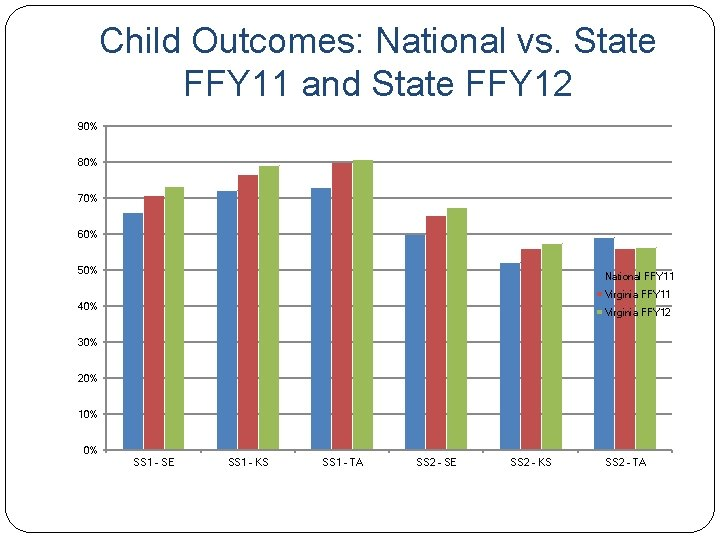Child Outcomes: National vs. State FFY 11 and State FFY 12 90% 80% 70% 60% 50% National FFY 11 Virginia FFY 11 40% Virginia FFY 12 30% 20% 10% 0% SS 1 - SE SS 1 - KS SS 1 - TA SS 2 - SE SS 2 - KS SS 2 - TA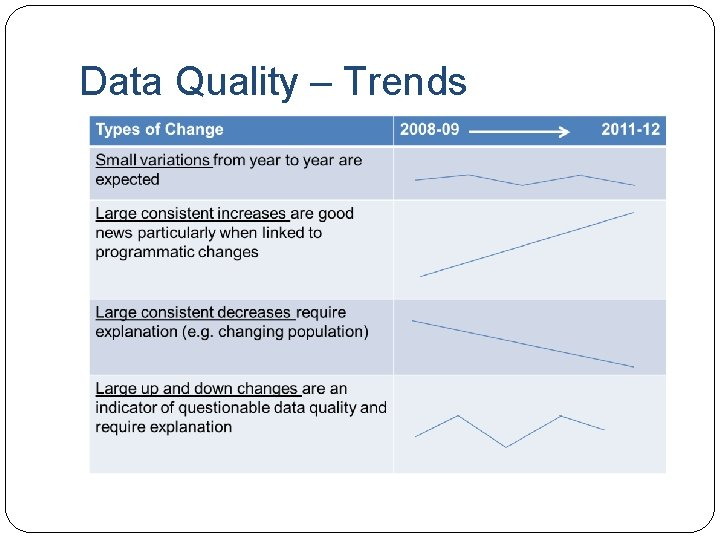Data Quality – Trends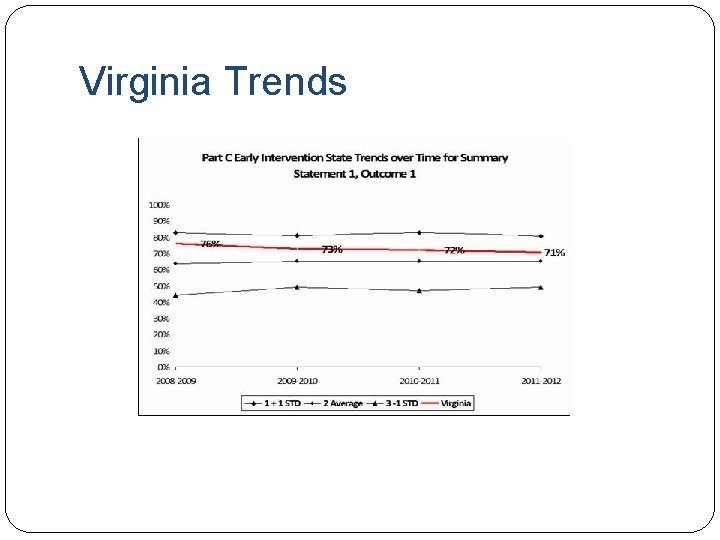Virginia Trends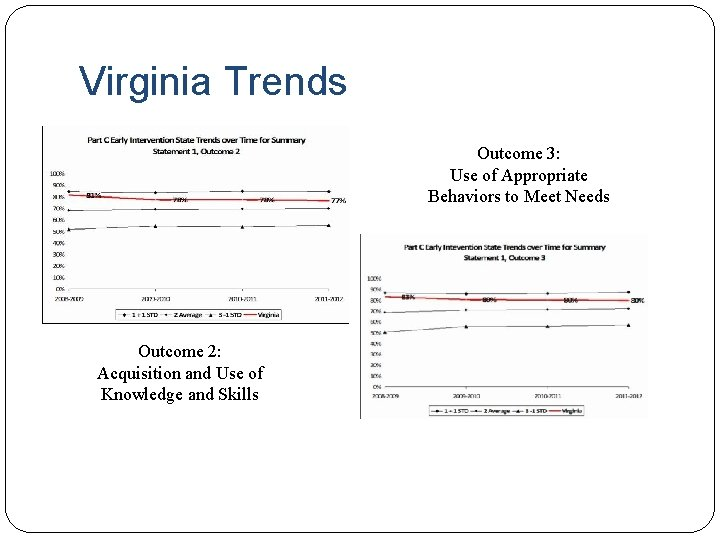Virginia Trends Outcome 3: Use of Appropriate Behaviors to Meet Needs Outcome 2: Acquisition and Use of Knowledge and Skills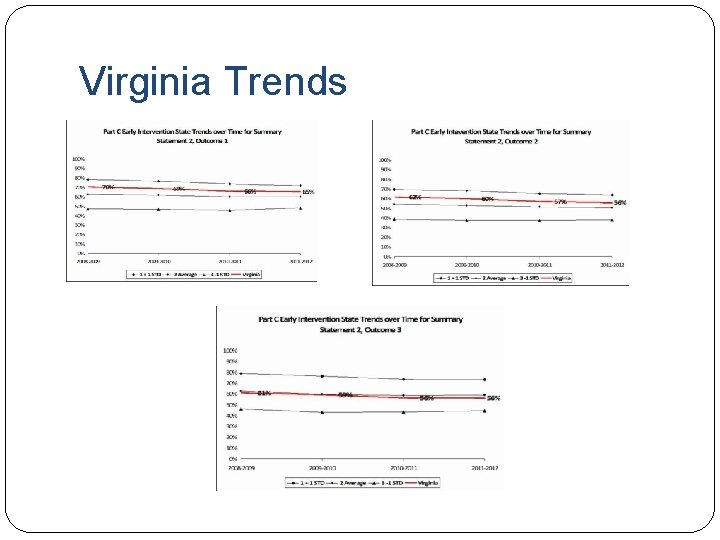Virginia Trends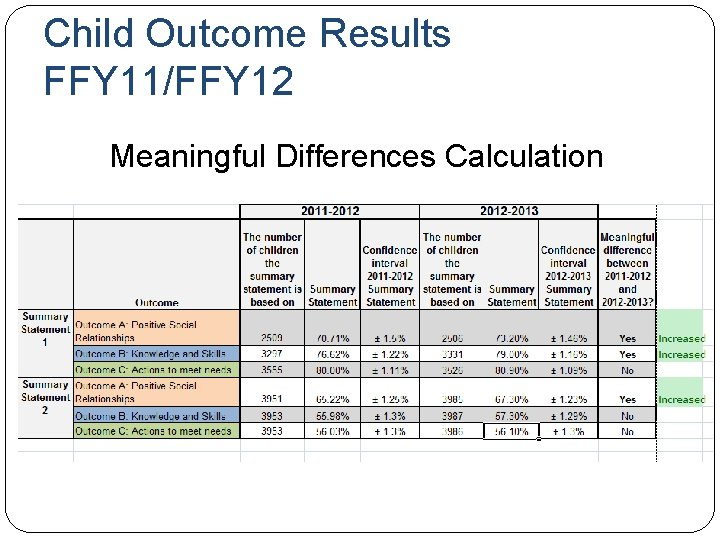Child Outcome Results FFY 11/FFY 12 Meaningful Differences CalculationChild Outcomes: National vs. State FFY 11 and State FFY 12 90% 80% 70% 60% 50% National FFY 11 Virginia FFY 11 40% Virginia FFY 12 30% 20% 10% 0% SS 1 - SE SS 1 - KS SS 1 - TA SS 2 - SE SS 2 - KS SS 2 - TA90% State (n=2509) 100% NN (n=7)* KK (n=37)* HH (n=46)* A (n=22)* R (n=84) MM (n=27) DD (n=125)* LL (n=52)* JJ (n=61)* C (n=32) II (n=107)* S (n=102)* V (n=90)* EE (n=14) FF (n=146)* W (n=124)* U (n=164)* X (n=129)* J (n=35) L (n=70) GG (n=113) B (n=100) O (n=181) I (n=122) D (n=39) N (n=64) M (n=114) E (n=32) K (n=79) Y (n=556)* BB (n=46) Q (n=133)* G (n=125)* AA (n=40)* F (n=48)* H (n=28) CC (n=33)* Z (n=40)* P (n=135)* T (n=24)* Child Outcomes: Local vs. State FFY 2012: Positive Social Relationships, Greater than Expected Growth 80% 70% 60% 50% 40% 30% 20% 10% 0%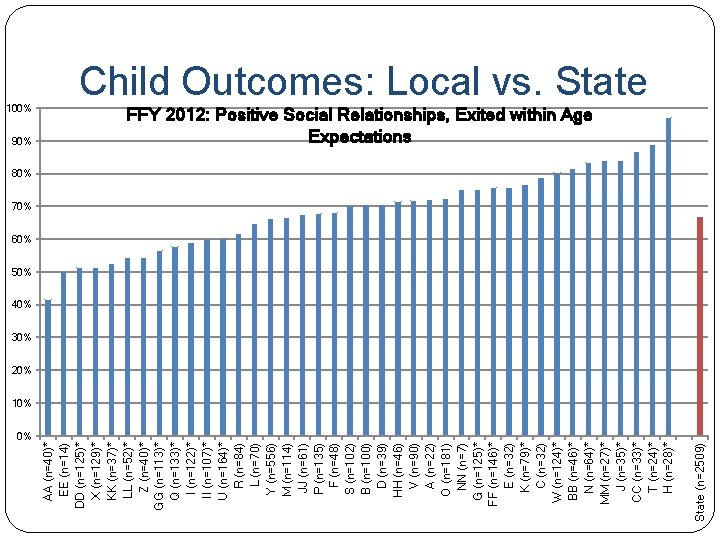State (n=2509) 90% AA (n=40)* EE (n=14) DD (n=125)* X (n=129)* KK (n=37)* LL (n=52)* Z (n=40)* GG (n=113)* Q (n=133)* I (n=122)* II (n=107)* U (n=164)* R (n=84) L (n=70) Y (n=556) M (n=114) JJ (n=61) P (n=135) F (n=48) S (n=102) B (n=100) D (n=39) HH (n=46) V (n=90) A (n=22) O (n=181) NN (n=7) G (n=125)* FF (n=146)* E (n=32) K (n=79)* C (n=32) W (n=124)* BB (n=46)* N (n=64)* MM (n=27)* J (n=35)* CC (n=33)* T (n=24)* H (n=28)* 100% Child Outcomes: Local vs. State FFY 2012: Positive Social Relationships, Exited within Age Expectations 80% 70% 60% 50% 40% 30% 20% 10% 0%State (n=3297) 100% NN (n=7)* KK (n=37)* JJ (n=61)* A (n=22) LL (n=52)* HH (n=46)* W (n=124)* DD (n=125)* MM (n=27) X (n=129)* R (n=84)* II (n=107)* FF (n=146) C (n=32) Q (n=133) S (n=102) L (n=70) GG (n=113) BB (n=46) I (n=122) U (n=164) V (n=90) B (n=100) D (n=39) Z (n=40) M (n=114) G (n=125) O (n=181) E (n=32) J (n=35) H (n=28) Y (n=556)* CC (n=33)* K (n=79)* N (n=64)* AA (n=40)* P (n=135)* T (n=24) F (n=48)* EE (n=14)* Child Outcomes: Local vs. State FFY 2012: Knowledge & Skills, Greater than Expected Growth 90% 80% 70% 60% 50% 40% 30% 20% 10% 0%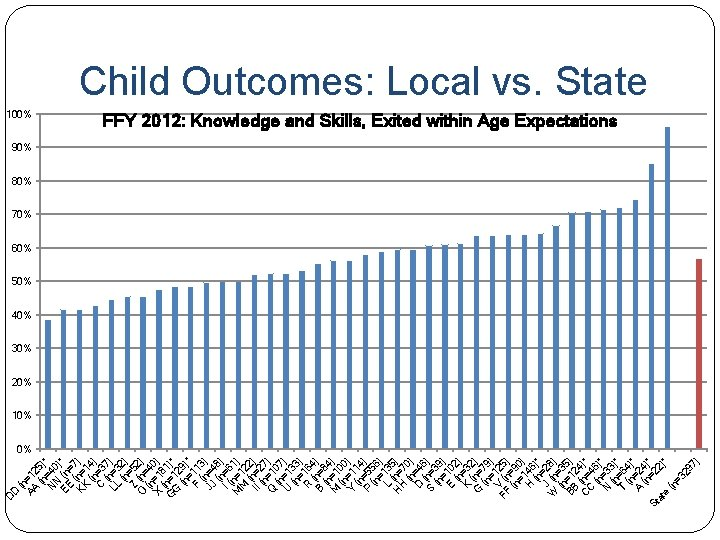(n AA =1 2 (n 5)* = N 40 N EE (n )* =7 KK (n= ) 1 (n 4) = C 3 ( 7 LL n=3 ) (n 2) Z =52 O (n= ) (n 4 X =1 0) ( 8 G n=1 1)* G 2 (n 9) = * F 113 (n ) JJ =4 (n 8) I ( =6 M n=1 1) M 2 ( 2 II n=2 ) (n 7 Q =1 ) (n 07 U =1 ) (n 33 = ) R 16 (n 4) B =8 (n 4 M =1 ) (n 00 Y =11 ) (n 4 P =5 ) (n 56 = ) L 135 ( H n= ) H 7 (n 0) D =4 ( 6 S n=3 ) (n 9 = ) E 10 (n 2) K =3 2 G (n= ) 7 (n 9 = ) V 12 FF (n 5) (n =9 =1 0) H 46 (n )* J =28 W (n ) (n =3 BB =1 5) 24 C (n= )* C 4 (n 6) N =3 * (n 3) T =64 * (n ) A =2 * (n 4)* =2 St 2) at * e (n =3 29 7) D D Child Outcomes: Local vs. State 100% FFY 2012: Knowledge and Skills, Exited within Age Expectations 90% 80% 70% 60% 50% 40% 30% 20% 10% 0%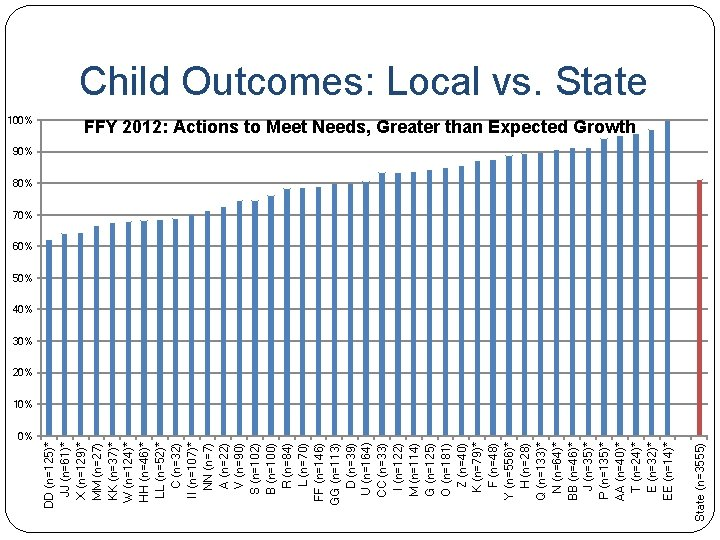State (n=3555) 100% DD (n=125)* JJ (n=61)* X (n=129)* MM (n=27) KK (n=37)* W (n=124)* HH (n=46)* LL (n=52)* C (n=32) II (n=107)* NN (n=7) A (n=22) V (n=90) S (n=102) B (n=100) R (n=84) L (n=70) FF (n=146) GG (n=113) D (n=39) U (n=164) CC (n=33) I (n=122) M (n=114) G (n=125) O (n=181) Z (n=40) K (n=79)* F (n=48) Y (n=556)* H (n=28) Q (n=133)* N (n=64)* BB (n=46)* J (n=35)* P (n=135)* AA (n=40)* T (n=24)* E (n=32)* EE (n=14)* Child Outcomes: Local vs. State FFY 2012: Actions to Meet Needs, Greater than Expected Growth 90% 80% 70% 60% 50% 40% 30% 20% 10% 0%(n F =40 (n )* = C 48 O (n= )* (n 3 2 EE =18 ) D ( 1)* D n= (n 1 =1 4) Z 25 ( ) LL n=4 * G ( 0 G n= ) (n 5 X =1 2) (n 13 U =1 )* (n 29 = ) P 16 * (n 4) KK =1 * 3 (n 5) I ( =3 n 7 II =12 ) (n 2 M =1 ) (n 07 H =1 ) H 1 (n 4) L =46 ( JJ n=7 ) 0 Q (n= ) (n 61 B =1 ) (n 33 = ) R 10 (n 0) D =8 (n 4) V =39 ( Y n= ) (n 90 S =5 ) ( 5 G n=1 6) (n 0 FF = 2) 1 ( 2 W n=1 5)* (n 46 =1 )* E 24 ( ) K n=3 * ( M n= 2) M 7 (n 9) H =2 * 7 C (n= )* C 2 (n 8) T =33 * (n )* N =24 N BB (n )* (n =7) J =46 (n ) A =3 * (n 5) N =2 * (n 2)* =6 St 4) at * e (n =3 55 5) AA 100% Child Outcomes: Local vs. State FFY 2012: Actions to Meet Needs, Exited within Age Expectations 90% 80% 70% 60% 50% 40% 30% 20% 10% 0%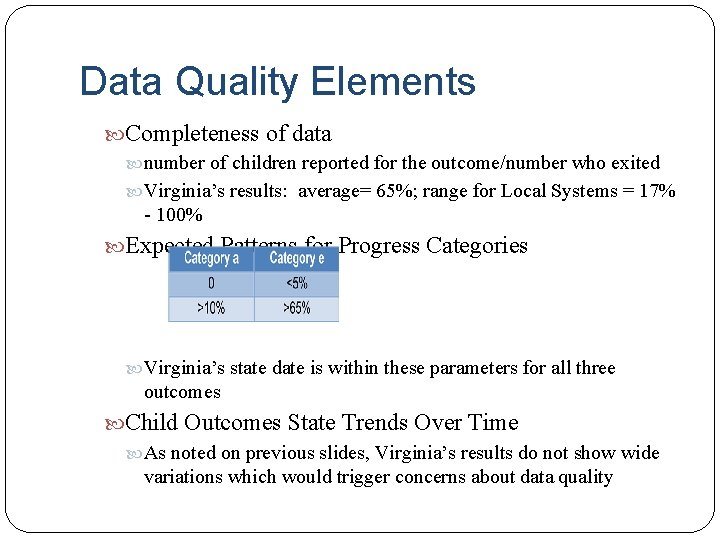Data Quality Elements Completeness of data number of children reported for the outcome/number who exited Virginia’s results: average= 65%; range for Local Systems = 17% - 100% Expected Patterns for Progress Categories Virginia’s state date is within these parameters for all three outcomes Child Outcomes State Trends Over Time As noted on previous slides, Virginia’s results do not show wide variations which would trigger concerns about data quality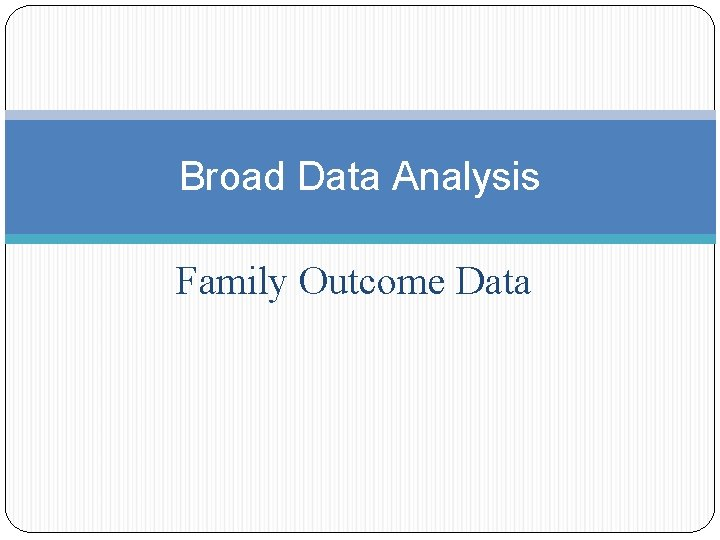Broad Data Analysis Family Outcome DataBroad Analysis: Family Outcomes Does our state’s data look different than the national data? Are our state family outcomes trends stable over time? Is the data trending upwards? Is the data trending downwards? Is our state performing more poorly in some outcomes than others? Are the family outcomes similar across programs? What about data quality? Can we be confident in our data?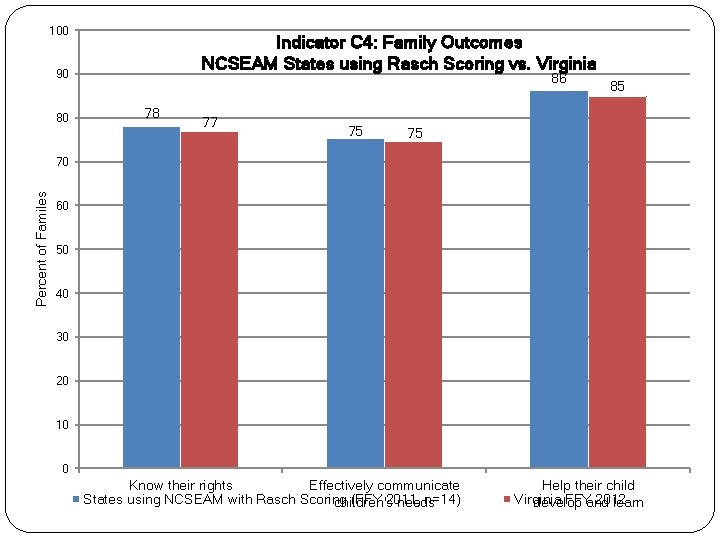100 Indicator C 4: Family Outcomes NCSEAM States using Rasch Scoring vs. Virginia 90 80 86 78 77 75 85 75 Percent of Familes 70 60 50 40 30 20 10 0 Know their rights Effectively communicate States using NCSEAM with Rasch Scoring (FFY 2011, n=14) children's needs Help their child Virginia FFYand 2012 develop learn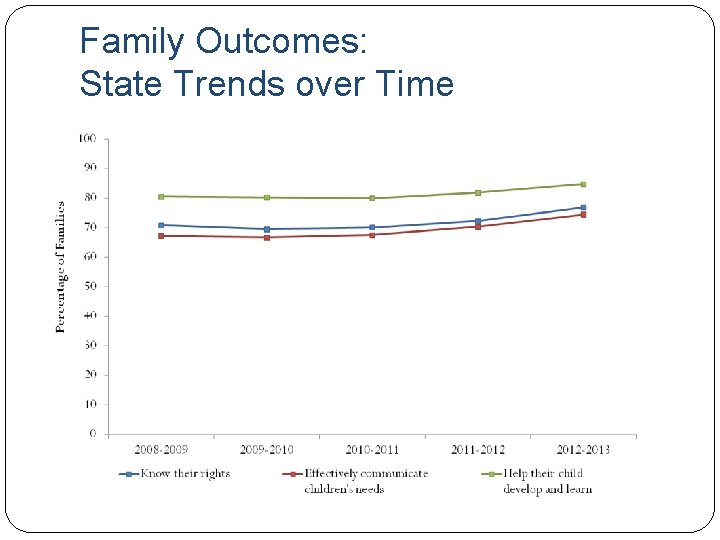Family Outcomes: State Trends over Time100 Indicator C 4: Family Outcomes NCSEAM States using Rasch Scoring vs. Virginia 90 80 86 78 77 75 85 75 Percent of Familes 70 60 50 40 30 20 10 0 Know their rights Effectively communicate States using NCSEAM with Rasch Scoring (FFY 2011, n=14) children's needs Help their child Virginia FFYand 2012 develop learn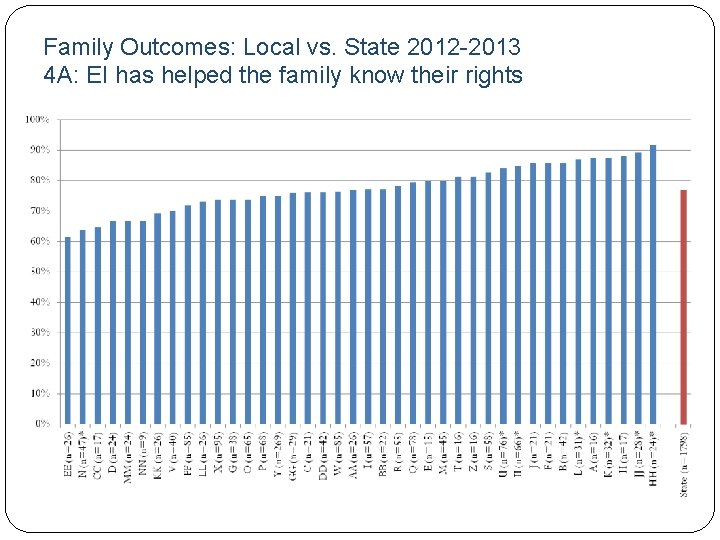Family Outcomes: Local vs. State 2012 -2013 4 A: EI has helped the family know their rightsFamily Outcomes: Local vs. State 2012 -2013 4 B: EI has helped the family communicate their children’s needsFamily Outcomes: Local vs. State 2012 -2013 4 C: EI has helped the family help their child develop and learnData Quality The data analysis for Virginia’ survey is quite extensive, using rigorous data analysis standards. Virginia’s response rate, like other states that use mailing as the means to disseminate the family survey, is lower than states who use other mechanisms.Broad Infrastructure Analysis Governan ce Monitoring and Accountabili ty Fiscal Broad Infrastructure Analysis Technical Assistanc e Quality Standards Data Profession al Developme nt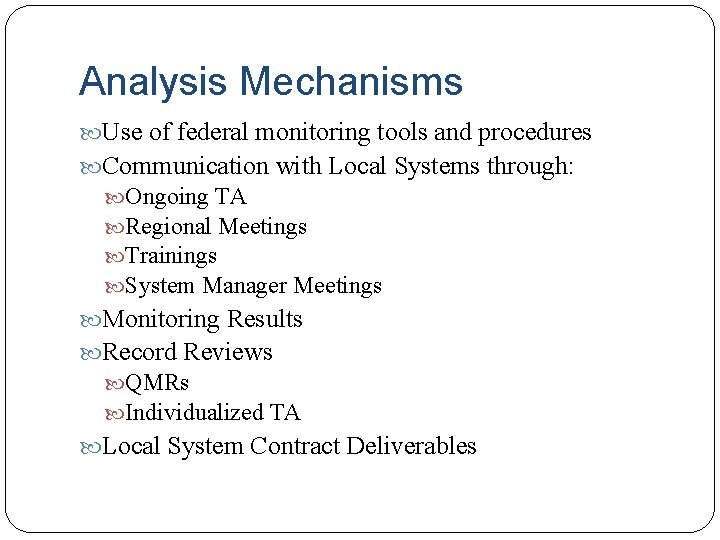Analysis Mechanisms Use of federal monitoring tools and procedures Communication with Local Systems through: Ongoing TA Regional Meetings Trainings System Manager Meetings Monitoring Results Record Reviews QMRs Individualized TA Local System Contract DeliverablesInfrastructure Analysis: Information Sources Local self-reporting Stakeholder input LSM surveys Training/meeting evaluations Monitoring/QMR Dispute Resolutions Observations through TA and other interactions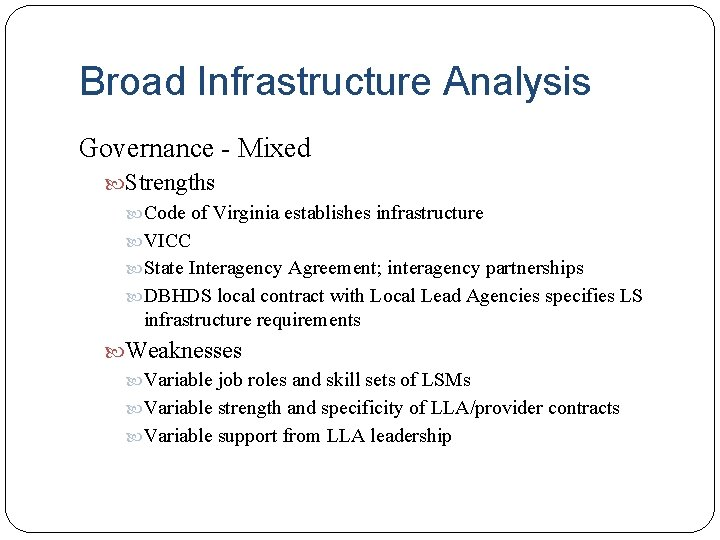Broad Infrastructure Analysis Governance - Mixed Strengths Code of Virginia establishes infrastructure VICC State Interagency Agreement; interagency partnerships DBHDS local contract with Local Lead Agencies specifies LS infrastructure requirements Weaknesses Variable job roles and skill sets of LSMs Variable strength and specificity of LLA/provider contracts Variable support from LLA leadership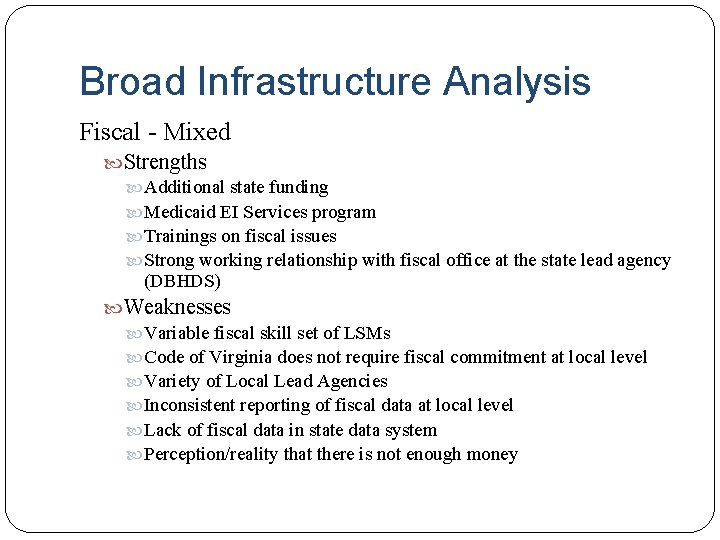Broad Infrastructure Analysis Fiscal - Mixed Strengths Additional state funding Medicaid EI Services program Trainings on fiscal issues Strong working relationship with fiscal office at the state lead agency (DBHDS) Weaknesses Variable fiscal skill set of LSMs Code of Virginia does not require fiscal commitment at local level Variety of Local Lead Agencies Inconsistent reporting of fiscal data at local level Lack of fiscal data in state data system Perception/reality that there is not enough moneyBroad Infrastructure Analysis Quality Standards – Not sure Practice Manual articulates expected practices, but these are not labeled quality practices Monitoring and Accountability – Strength Local self-monitoring and supervision for continuous improvement – variable Professional Development – Strength Technical Assistance – Strength Data – WeaknessClosing Thoughts Based on this broad review, did anything strike you as an area of focus for our Systemic Improvement Plan?